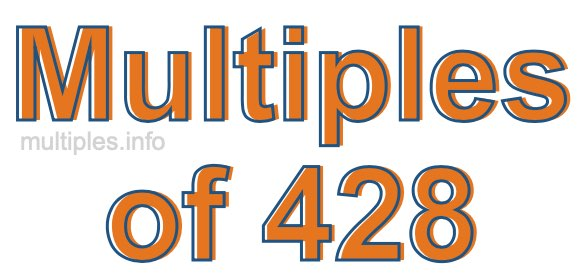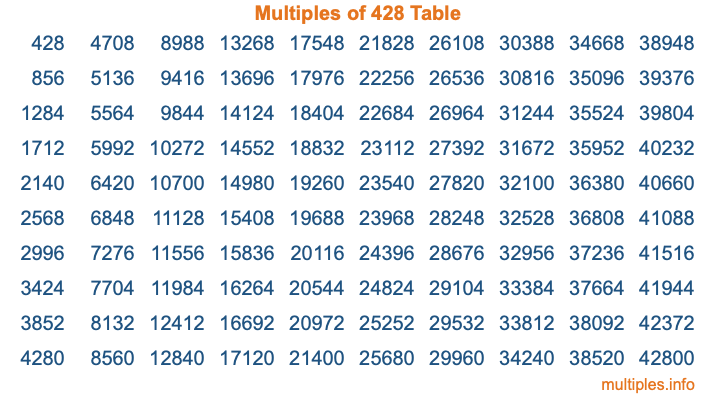Multiples of 428Welcome to the Multiples of 428 page. Here we will first teach you everything you will ever need to know about the multiples of 428, and then give you a study guide summary of everything we taught you to make sure you remember it all. Use this page to look up facts and learn information about the multiples of 428. This page will make you a multiples of four hundred twenty-eight expert!

Definition of Multiples of 428
Multiples of 428 are all the numbers that when divided by 428 equal an integer. Each of the multiples of 428 are called a multiple. A multiple of 428 is created by multiplying 428 by an integer.

Therefore, to create a list of multiples of 428, you start with 1 multiplied by 428, then 2 multiplied by 428, then 3 multiplied by 428, and so on for as long as you want. Thus, the list of the first five multiples of 428 is 428, 856, 1284, 1712, and 2140. To see a larger list of multiples of 428, see the printable image of Multiples of 428 further down on this page. We also have a category where you can choose any nth multiple of 428.

Multiples of 428 Checker
The Multiples of 428 Checker below checks to see if any number of your choice is a multiple of 428. In other words, it checks to see if there is any number (integer) that when multiplied by 428 will equal your number. To do that, we divide your number by 428. If the the quotient is an integer, then your number is a multiple of 428.

Is  a multiple of 428?

Least Common Multiple of 428 and ...
A Least Common Multiple (LCM) is the lowest multiple that two or more numbers have in common. This is also called the smallest common multiple or lowest common multiple and is useful to know when you are adding our subtracting fractions. Enter one or more numbers below (428 is already entered) to find the LCM.

Check out our LCM Calculator if you need more details about the Least Common Multiple or if you need the LCM for different numbers for adding and subtraction fractions.

nth Multiple of 428
As we stated above, 428 is the first multiple of 428, 856 is the second multiple of 428, 1284 is the third multiple of 428, and so on. Enter a number below to find the nth multiple of 428.

th multiple of 428

Multiples of 428 vs Factors of 428
428 is a multiple of 428 and a factor of 428, but that is where the similarities end. All postive multiples of 428 are 428 or greater than 428. All positive factors of 428 are 428 or less than 428.

Below is the beginning list of multiples of 428 and the factors of 428 so you can compare:

Multiples of 428: 428, 856, 1284, 1712, 2140, etc.

Factors of 428: 1, 2, 4, 107, 214, 428

As you can see, the multiples of 428 are all the numbers that you can divide by 428 to get a whole number. The factors of 428, on the other hand, are all the whole numbers that you can multiply by another whole number to get 428.

It's also interesting to note that if a number (x) is a factor of 428, then 428 will also be a multiple of that number (x).

Multiples of 428 vs Divisors of 428
The divisors of 428 are all the integers that 428 can be divided by evenly. Below is a list of the divisors of 428.

Divisors of 428: 1, 2, 4, 107, 214, 428

The interesting thing to note here is that if you take any multiple of 428 and divide it by a divisor of 428, you will see that the quotient is an integer.

Multiples of 428 Table
Below is an image of the first 100 multiples of 428 in a table. The table is in chronological order, column by column. The first column has the first ten multiples of 428, the second column has the next ten multiples of 428, and so on.The Multiples of 428 Table is also referred to as the 428 Times Table or Times Table of 428. You are welcome to print out our table for your studies.

Negative Multiples of 428
Although not often discussed or needed in math, it is worth mentioning that you can make a list of negative multiples of 428 by multiplying 428 by -1, then by -2, then by -3, and so on, to get the following list of negative multiples of 428:

-428, -856, -1284, -1712, -2140, etc.

Multiples of 428 Summary
Below is a summary of important Multiples of 428 facts that we have discussed on this page. To retain the knowledge on this page, we recommend that you read through the summary and explain to yourself or a study partner why they hold true.

There are an infinite number of multiples of 428.

A multiple of 428 divided by 428 will equal a whole number.

428 divided by a factor of 428 equals a divisor of 428.

The nth multiple of 428 is n times 428.

The largest factor of 428 is equal to the first positive multiple of 428.

428 is a multiple of every factor of 428.

428 is a multiple of 428.

A multiple of 428 divided by a divisor of 428 equals an integer.

428 divided by a divisor of 428 equals a factor of 428.

Any integer times 428 will equal a multiple of 428.

Multiples of a Number
Here you can get the multiples of another number, all with the same attention to detail as we did for multiples of 428 on this page.

Multiples of
Multiples of 429
Did you find our page about multiples of four hundred twenty-eight educational? Do you want more knowledge? Check out the multiples of the next number on our list!